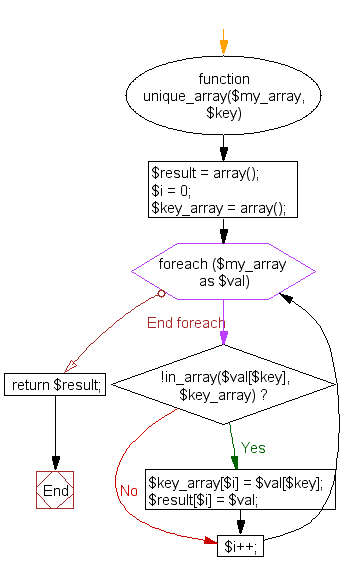﻿ PHP Array Exercise: Create a multidimensional unique array for any single key index - w3resource# PHP Array Exercises : Create a multidimensional unique array for any single key index

## PHP Array: Exercise-38 with Solution

Write a PHP function to create a multidimensional unique array for any single key index.

Sample Solution:

PHP Code:

``````<?php
function unique_array(\$my_array, \$key) {
\$result = array();
\$i = 0;
\$key_array = array();

foreach(\$my_array as \$val) {
if (!in_array(\$val[\$key], \$key_array)) {
\$key_array[\$i] = \$val[\$key];
\$result[\$i] = \$val;
}
\$i++;
}
return \$result;
}

\$students = array(
0 => array("city_id"=>"1", "name"=>"Sara",  "mobile_num"=>"1111111111"),
1 => array("city_id"=>"2", "name"=>"Robin", "mobile_num"=>"2222222222"),
2 => array("city_id"=>"1", "name"=>"Sonia", "mobile_num"=>"3333333333"),
);

print_r(unique_array(\$students, "city_id"));
?>
```
```

Sample Output:

```Array
(
 => Array
(
[city_id] => 1
[name] => Sara
[mobile_num] => 1111111111
)

 => Array
(
[city_id] => 2
[name] => Robin
[mobile_num] => 2222222222
)

)
```

Flowchart:PHP Code Editor:

What is the difficulty level of this exercise?

﻿

## PHP: Tips of the Day

PHP: Extract numbers from a string

```\$str = 'In My Cart : 11 12 items';
preg_match_all('!\d+!', \$str, \$matches);
print_r(\$matches);
```